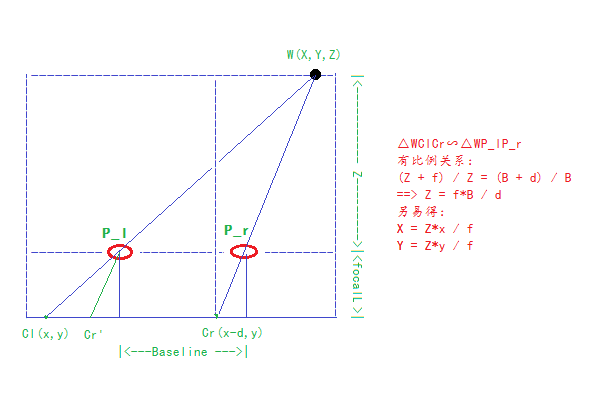基于OpenCV的立体相机标定StereoCalibration与目标三维坐标定位

1.在做立体相机标定的时候，标定板的规范与否直接影响到最后标定的结果，进而会影响目标3D坐标重建。

2.立体相机标定的一些概念

2.1.相机投影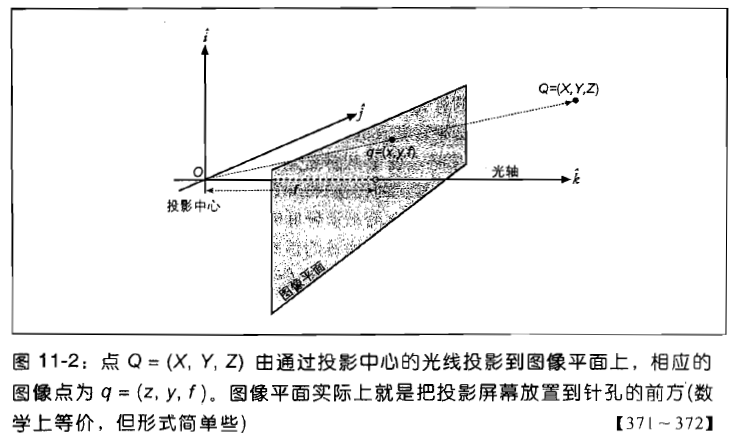x = fx(X/Z)+cx,y = fy(Y/Z) + cy;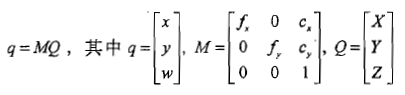2.2.透镜畸变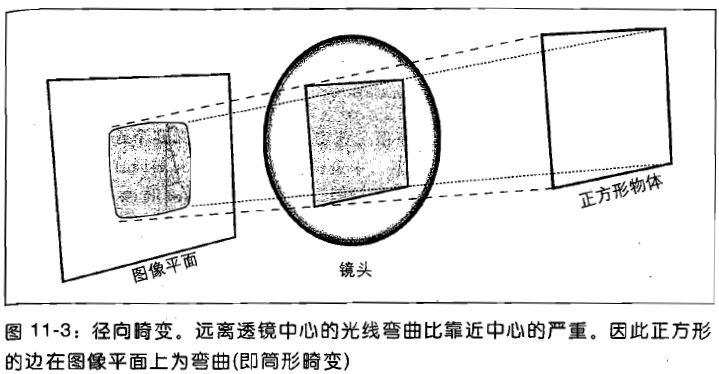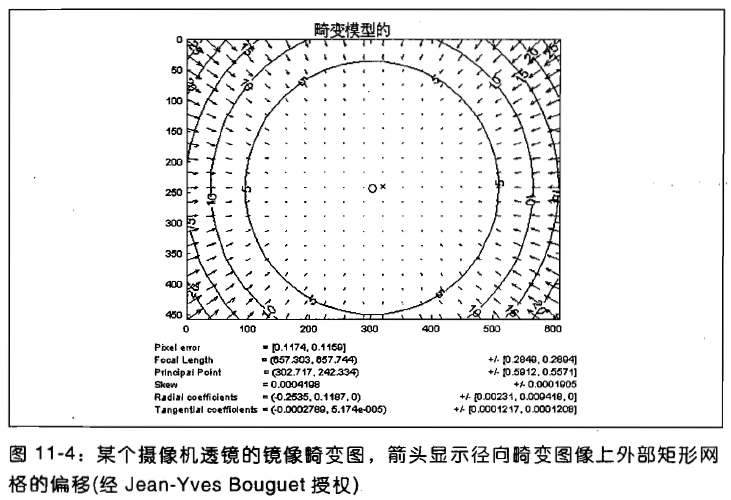x_corrected = x(1+k1*r²+k2*r^4+k3*r^6);

y_corrected = y(1+k1*r²+k2*r^4+k3*r^6).

(x,y)为畸变点在成像仪上的原始位置，(x_corrected,y_corrected)为矫正后的新位置，标定中，k1,k2,k3为畸变内参。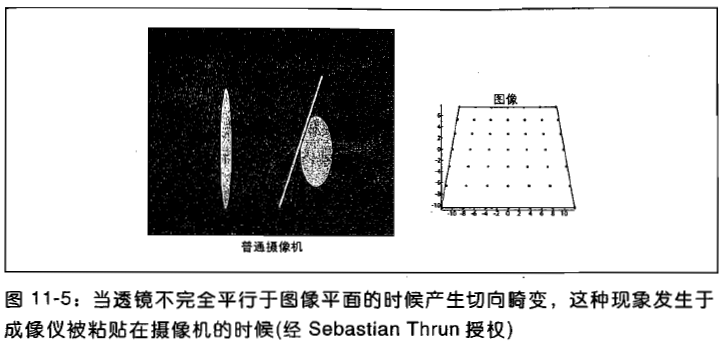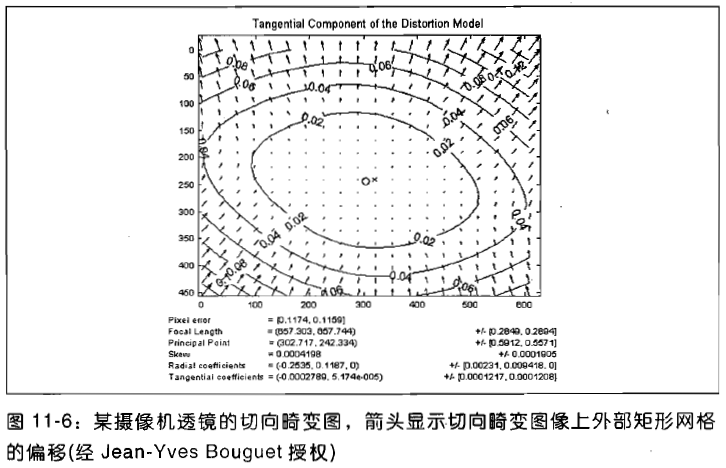x_corrected = x + [2*p1*y+p2*(r^2+2x^2)]

y_corrected = y + [p1*(r^2+2*y^2)+2*p2*x]

2.3.立体成像stereo imaging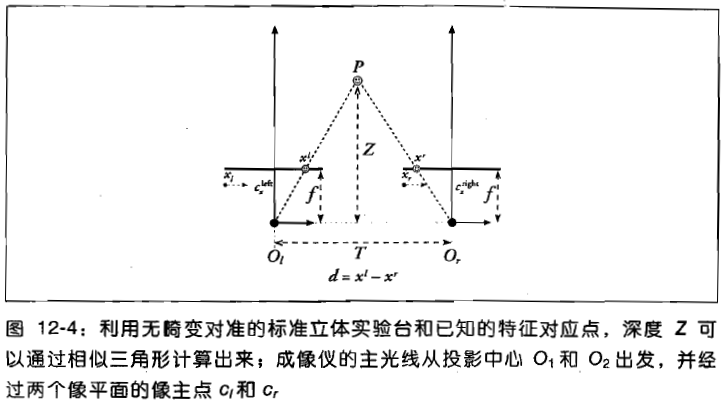T-(xl-xr) : (Z-f) = T : Z ==> Z = f*T/(xl - xr)

f为焦距，T为baseline，(xl - xr)为视差，可知求取深度信息只与这三个值有关。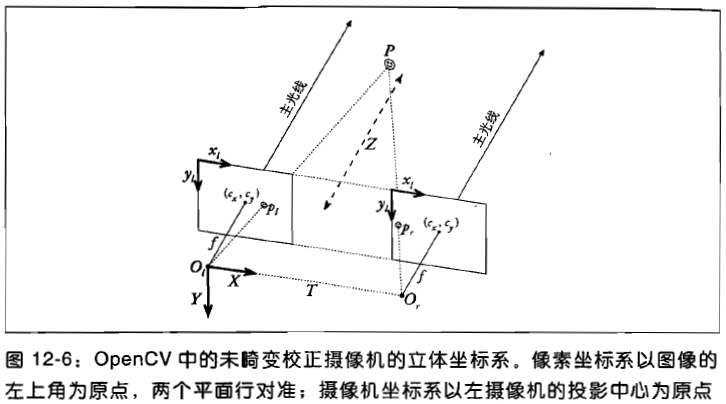2.4.对极几何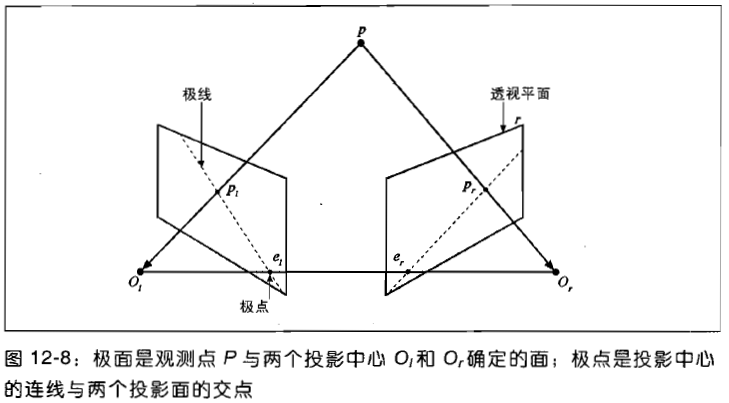1.摄像机视图内的每个3D点都包含在极面内，极面与每幅图像相交的直线是极线，

2.指定一个图像上面的特征，它在另一幅图像上的匹配视图一定在对应的极线上，这就是“对极约束”，

3.有了对极约束之后，意味着对二幅图像间特征匹配的二维搜索转变成了沿着极线的一维搜索，这不仅节约了时间，还可排除大量的虚假匹配，

4.不同特征的次序时保留的，如果A,B在两成像仪中都可见，按顺序水平出现，那么在另一成像仪上也是水平出现。

2.5.本征矩阵E与基础矩阵F

2.6.重投影矩阵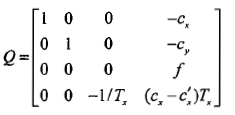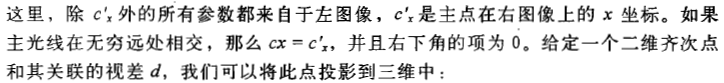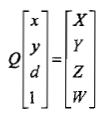3.立体标定stereo calibration

3.1.输入立体图像对文件：stereo_images.xml

<?xml version="1.0"?>

<opencv_storage>

<imagelist>

"left01.bmp" "left02.bmp" ...

</imagelist>

</opencv_storage>

3.2.输入squareSize:实际的棋盘格大小mm

3.3输出文件：intrinsic.yml, extrinsic.yml

4.参数解释

intrinsic params内参

M1,M2--内参矩阵

[fx  0  cx;

M = 0  fy   cy;

0  0   1]

D1,D2--畸变向量

D =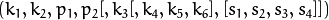k为径向畸变，p为切向畸变.

extrinsic params外参

R--右相机相对左相机的旋转矩阵

T--右相机相对左相机的平移矩阵

R1,R2--左右相机校准变换（旋转）矩阵

P1,P2--左右相机在校准后坐标系中的投影矩阵

Q--视差-深度映射矩阵，我利用它来计算单个目标点的三维坐标

5.三维坐标计算

step1. 立体相机标定，获取相机系统内外参数

step2. 获取目标点在左右成像平面上的像素坐标

step3. 利用标定参数对像素坐标进行校准undistortPoints

step4. 利用重投影矩阵计算目标三维坐标